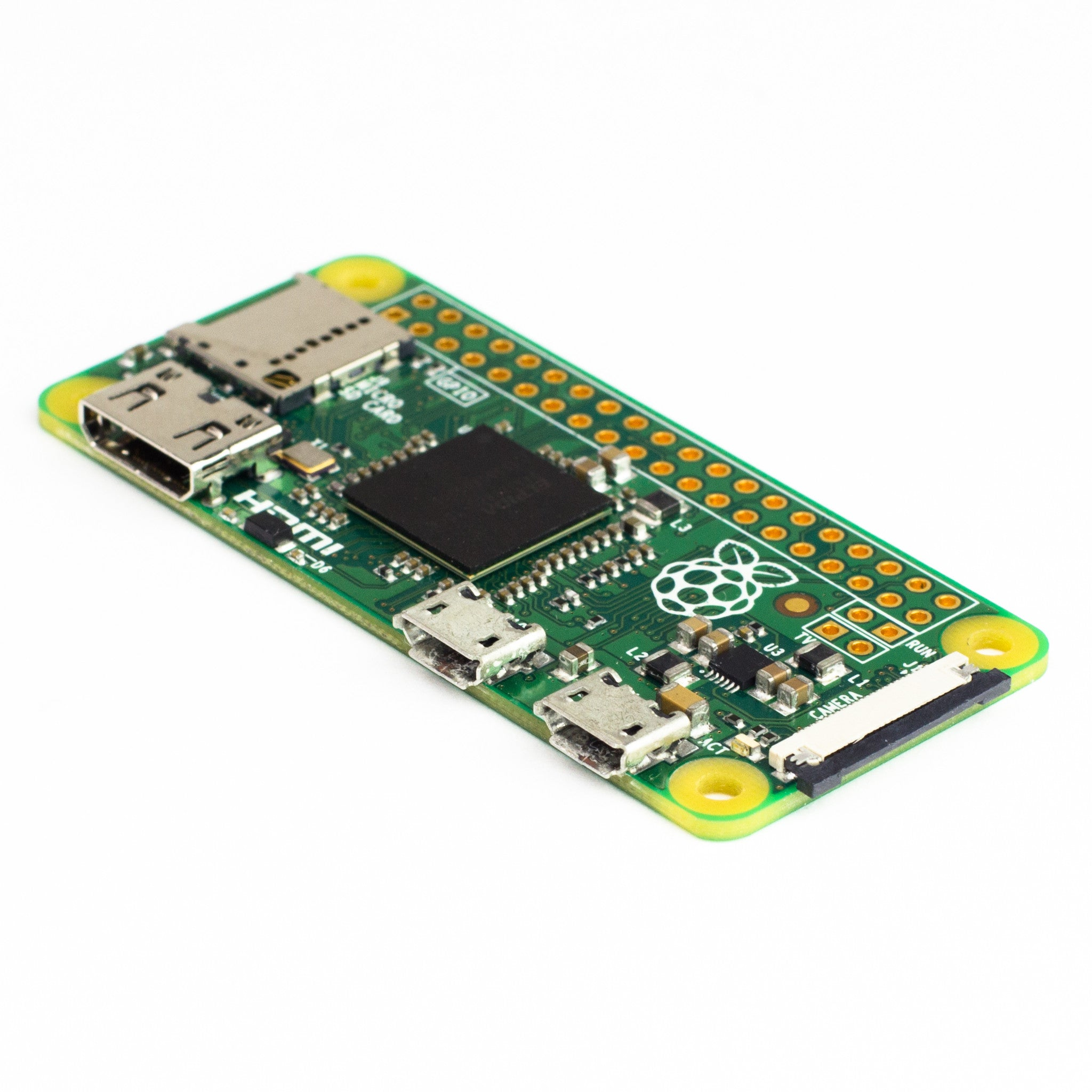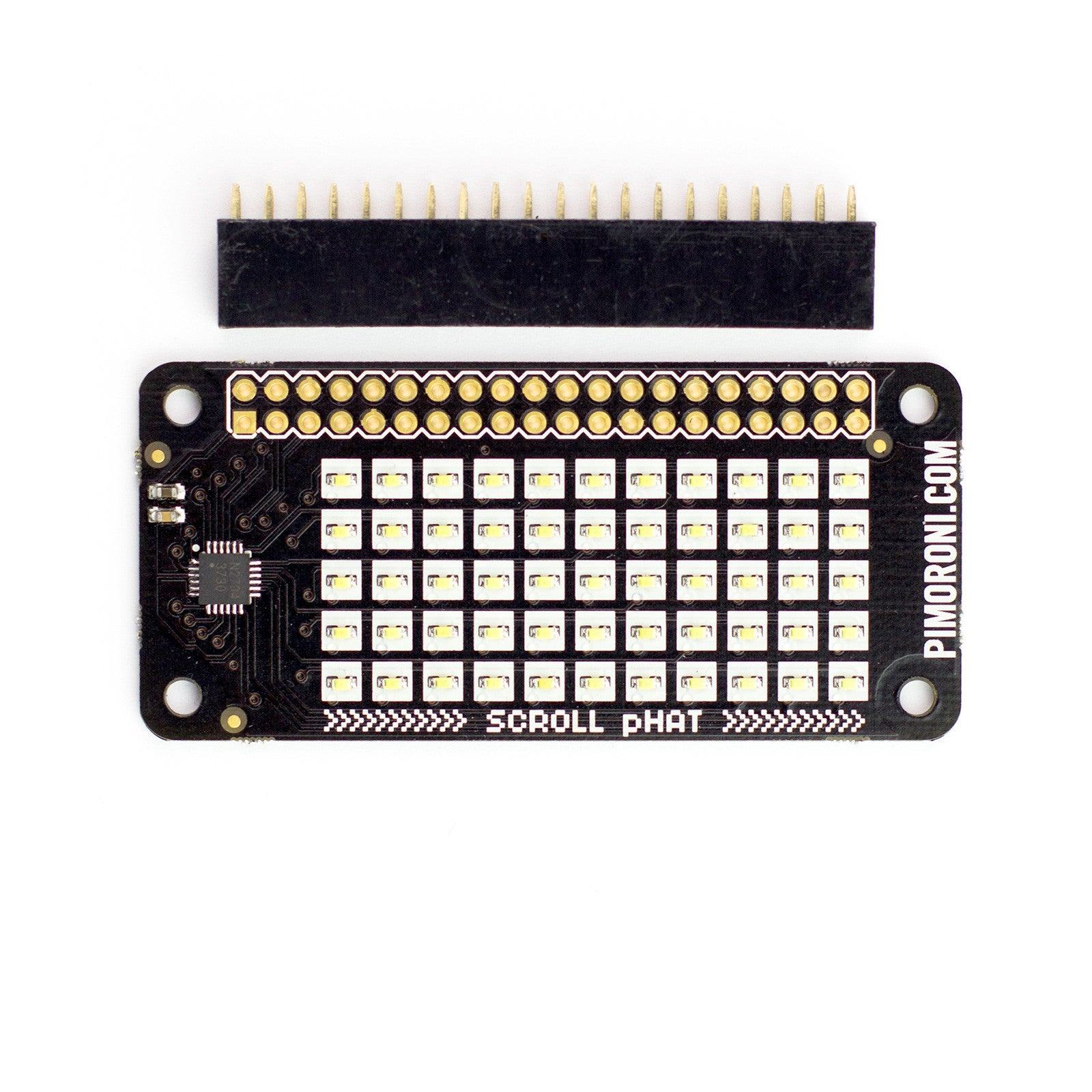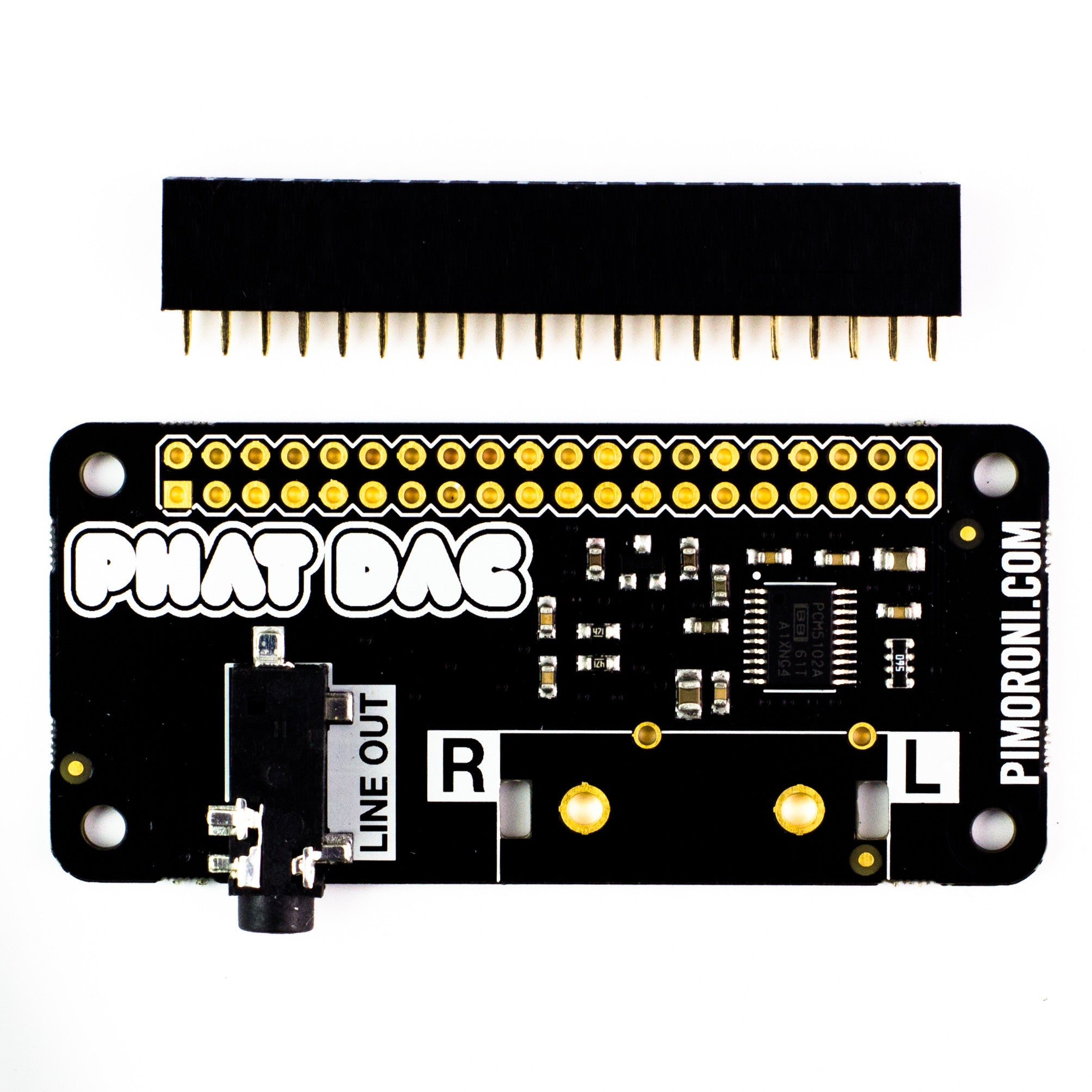## Build a spectrum analyser with Scroll pHAT and pHAT DACThis tutorial will show you how to set up a tiny little spectrum analyser with a Raspberry Pi Zero, a pHAT DAC (digital to analogue converter) and a Scroll pHAT LED matrix. We'll walk through how to solder the pHATs, how to install the necessary software and then how the code itself works.

What you'll need:

• A Raspberry Pi Zero
• A pHAT DAC
• A Scroll pHAT
• A male 40 pin header
• An extended female 40 pin header
• A soldering iron with a conical/pointed tip
• A roll of masking tape (recommended, but not essential)
• Panavise (recommended, but not essential)

## Soldering the Pi Zero and pHATs

Since the Pi Zero and the pHAT come un-soldered, you'll need to solder headers onto them first. We've written a little guide to show you how to solder headers onto pHATs, so we'd suggest that you refer to that if you're not already a dab hand with a soldering iron.

First, you'll want to solder a male 40 pin header onto the Pi Zero. Make sure that you solder it so that the longer ends of the pins are pointing up the way, and the shorter ends are protruding down through the holes on the Pi Zero where you'll solder them.

The pHATs will need to be stacked on top of each other, with the pHAT DAC below and the Scroll pHAT on top. There are a couple of ways to do this.

If you want to use the Scroll pHAT for other things besides just this spectrum analyser, then you can just solder a normal 40 pin female header to it and solder an extended 40 pin female header to the pHAT DAC, meaning that you can mount the Scroll pHAT on the pHAT DAC in the usual way.

Or, you can do it more permanently by first soldering the extended female header to the pHAT DAC and then soldering the Scroll pHAT directly on top and clipping any extra bits of pin protruding out from the top afterwards.

We chose to do it the permanent way, with the extended female header, and you can see the two stages in the images below.## Setting up pHAT DAC

Make sure that you're starting with a fresh, up-to-date version of Raspbian, as this is what was used when putting together this tutorial and we can't vouch that it will work properly otherwise.

There's a handy one line installer for pHAT DAC that should get everything set up properly. In the terminal, type the following:

``````curl https://get.pimoroni.com/phatdac | bash
``````

The installer should prompt you to reboot but, if it doesn't, type in the terminal, `sudo reboot`.

You can test that it has worked properly by connecting a speaker to your pHAT DAC (make sure that you don't use headphones, as pHAT DAC is designed for line level output) and typing:

``````aplay /usr/share/sounds/alsa/Front_Center.wav
``````

We also need to install the `alsaaudio` Python library. Do this by typing:

``````sudo apt-get install python-alsaaudio
``````

Assuming that all worked, we'll move on to getting Scroll pHAT installed and working.

## Setting up Scroll pHAT

There's also a one line installer for Scroll pHAT. Type the following in the terminal to install the Scroll pHAT library:

``````curl https://get.pimoroni.com/scrollphat | bash
``````

Again, it should prompt you to reboot but, if it doesn't, type `sudo reboot` in the terminal.

You can test that it worked by typing, in the terminal:

``````sudo python scroll-phat/examples/cpu.py
``````

This should graph out your CPU usage, scrolling from right to left.

## Fast Fourier Transform (FFT)

The Fast Fourier Transform is an incredibly nifty way of converting values from the time domain into a spectrum of different frequencies for a given time period. In this way, we can average out, or compress, audio data in real time. The niftiest feature of FFT is how quick an algorithm it is for the amount of data processed. It's used commonly in image, video and audio compression, and you're probably taking advantage of it many times each day without even being aware of it.

Fortunately, there's a Python library, Numpy, that can do FFT, amongst many other things.

We can read in audio data in real time, use the FFT at a given sampling rate to compute the FFT for the whole audio spectrum, and then average it out into the number of bands we want - 11 - to fit onto Scroll pHAT's matrix.

## The code

### Imports

We'll do all of this in Python. We'd recommend that you put all of the following code into a text file, in your favourite text editor, and then save it as something like `spectrum_analyser.py`. Of course, you could do it all interactively in a Python prompt, but that'd be a real pain.

Once your code is complete, you can open a terminal and run your code by typing `sudo python spectrum_analyser.py`.

First, we'll import all of the libraries that we need for our little spectrum analyser program:

``````import sys
import wave
import alsaaudio as aa
from struct import unpack
import numpy as np
import scrollphat
``````

We'll need the `sys` library to parse the command line arguments passed to our script.

The `wave`, `alsaaudio`, `struct` and `numpy` libraries will be used to read in and analyse the audio we pass to our program.

Finally, we'll use the `scrollphat` library to display the audio data on our Scroll pHAT matrix.

### Settings

Below our imports, and before our functions, we'll set some settings that will determine how our audio is processed.

First, we'll open our `.wav` file, using the `wave` library, having grabbed the filename with `sys.argv` from the `sys` library:

``````wavfile = wave.open(sys.argv, 'r')
``````

Passing the filename as an argument is a better approach than hard-coding the path to the file, as we can change what file we pass on the fly. To run our program with a `.wav` file called `sailorshornpipe.wav` we'd type the following in the terminal once our code is complete:

``````sudo python spectrum_analyser.py sailorshornpipe.wav
``````

Note that it's `sys.argv` rather than `sys.argv`, because `sys.argv` refers to the program name itself.

Next, we'll set some parameters for the audio output. As well as processing the audio, we need to feed the audio back out, so that we can hear it as well as seeing it!

``````sample_rate = wavfile.getframerate()
no_channels = wavfile.getnchannels()
chunk = 4096

output = aa.PCM(aa.PCM_PLAYBACK, aa.PCM_NORMAL)
output.setchannels(no_channels)
output.setrate(sample_rate)
output.setformat(aa.PCM_FORMAT_S16_LE)
output.setperiodsize(chunk)
``````

We get the sample rate and number of channels from our `.wav` file, and set the chunk size, which determines how many chunks our audio is broken into for processing.

Then, we set up an alsa audio output and set the same parameters that we got from our `.wav` file.

We'll need a couple of lists in which to store our values that we get from the audio, and also a list of weightings that will be applied to each of our frequency bands.

``````matrix    = [0, 0, 0, 0, 0, 0, 0, 0, 0, 0, 0]
power     = []
weighting = [1, 1, 2, 4, 8, 8, 8, 8, 16, 16, 16]
``````

The `power` list will store values from our Fourier transform and the `matrix` list will store the actual values that we'll display on our spectrum. Because different frequency bands will be more prevalent than others, we weight each band differently, to even out the spectrum. This is what our `weighting` list does, and you can tweak these values if you like, to get your spectrum looking the way you want. These values worked well for us.

Lastly, we set the brightness of our Scroll pHAT a little below full brightness.

``````scrollphat.set_brightness(5)
``````

### The Fast Fourier Transform

We'll write two different functions to help with our Fast Fourier Transform.

The first is a simple function that takes a frequency value and returns its index (position) in our array of computed FFT values. This will allow us to average out each frequency band when we compute the FFT values.

``````def power_index(val):
return int(2 * chunk * val / sample_rate)
``````

The second is the function that will compute the FFT for each chunk of our audio data. Here's the complete function, and then we'll break it down step-by-step.

``````def compute_fft(data, chunk, sample_rate):
global matrix
data = unpack("%dh" % (len(data) / 2), data)
data = np.array(data, dtype='h')

fourier = np.fft.rfft(data)
fourier = np.delete(fourier, len(fourier) - 1)

power = np.abs(fourier)

matrix = int(np.mean(power[power_index(0)    :power_index(156) :1]))
matrix = int(np.mean(power[power_index(156)  :power_index(313) :1]))
matrix = int(np.mean(power[power_index(313)  :power_index(625) :1]))
matrix = int(np.mean(power[power_index(625)  :power_index(1000) :1]))
matrix = int(np.mean(power[power_index(1000) :power_index(2000) :1]))
matrix = int(np.mean(power[power_index(2000) :power_index(3000) :1]))
matrix = int(np.mean(power[power_index(3000) :power_index(4000) :1]))
matrix = int(np.mean(power[power_index(4000) :power_index(5000) :1]))
matrix = int(np.mean(power[power_index(5000) :power_index(6000) :1]))
matrix = int(np.mean(power[power_index(6000) :power_index(7000) :1]))
matrix = int(np.mean(power[power_index(7000):power_index(8000) :1]))

matrix = np.divide(np.multiply(matrix, weighting), 1000000)
matrix = matrix.clip(0, 5)
matrix = [float(m) for m in matrix]

return matrix
``````

We pass a number of different things into the function: the piece of audio data itself, the chunk size and the sample_rate.

``````compute_fft(data, chunk, sample_rate)
``````

Next, we declare `matrix` as a global variable, meaning that it exists outside the scope of this function.

We also unpack the binary C short type data to a type that Python can interpret and then convert it into a numpy array, ensuring that we tell it that the data is in the C short type - `dtype='h'`.

``````global matrix
data = unpack("%dh" % (len(data) / 2), data)
data = np.array(data, dtype='h')
``````

Next, we do the actual Fourier transform. We're using the `rfft` function from `numpy.fft` since we're using real data, and then we delete the last element in the array, so that our array size matches the chunk size.

``````fourier = np.fft.rfft(data)
fourier = np.delete(fourier, len(fourier) - 1)
``````

Because the Fourier transform will return positive and negative values, we take the absolute values of the numpy array, which converts all of the negative values to positive ones.

``````power = np.abs(fourier)
``````

We need to split our frequency spectrum up into 11 bands to display on our Scroll pHAT. To do that, we'll slice our `power` array of FFT-computed frequencies into 11 pieces using our `power_index` function, take the mean (average) of each piece, and then read them into our `matrix` list at the appropriate position.

``````matrix = int(np.mean(power[power_index(0)    :power_index(156) :1]))
matrix = int(np.mean(power[power_index(156)  :power_index(313) :1]))
matrix = int(np.mean(power[power_index(313)  :power_index(625) :1]))
matrix = int(np.mean(power[power_index(625)  :power_index(1000) :1]))
matrix = int(np.mean(power[power_index(1000) :power_index(2000) :1]))
matrix = int(np.mean(power[power_index(2000) :power_index(3000) :1]))
matrix = int(np.mean(power[power_index(3000) :power_index(4000) :1]))
matrix = int(np.mean(power[power_index(4000) :power_index(5000) :1]))
matrix = int(np.mean(power[power_index(5000) :power_index(6000) :1]))
matrix = int(np.mean(power[power_index(6000) :power_index(7000) :1]))
matrix = int(np.mean(power[power_index(7000):power_index(8000) :1]))
``````

Lastly, we multiply our matrix by our `weighting` list and divide by 1,000,000 to get the numbers into a more sensible scale. We'll also clip each of the 11 values to a maximum of 5, so that they can be displayed on Scroll pHAT's columns, which are 5 LEDs high, and then convert them to floats to make them play nice with Scoll pHAT's `graph` method.

``````matrix = np.divide(np.multiply(matrix, weighting), 1000000)
matrix = matrix.clip(0, 5)
matrix = [float(m) for m in matrix]
``````

### Processing the audio

Our `wavfile`, read in with the `wave` library, has a convenient `readframes` method that can read the audio data in chunks of a given size. We can simply use our `chunk` variable that we set earlier to tell it how big the chunks should be. Each time this function is called, it will parse another chunk of audio. Ideal for our purposes!

``````data = wavfile.readframes(chunk)
``````

Finally, we use a `while` loop to i) output the audio, ii) compute the FFT, iii) display the FFT bands on Scroll pHAT with its `graph` method, specifying the range (0-5) and, iv) to read the next chunk of data.

``````while data != '':
output.write(data)
matrix = compute_fft(data, chunk, sample_rate)
scrollphat.graph(matrix, 0, 5)
``````

### Running the example

As we mentioned above, you can run the example on your `wav` audio file by typing the following in a terminal, assuming your audio file is called `sailorshornpipe.wav`.

``````sudo python spectrum_analyser.py sailorshornpipe.wav
``````

Here's an example of how it should look.In theory, it's possible to stream audio data directly into a Python script, so you could stream all of the system audio through the script and display the spectra on Scroll pHAT, although that's beyond the scope of this tutorial. We'd encourage you to give it a shot though.

There's a complete copy of the code below.

``````import sys
import wave
import alsaaudio as aa
from struct import unpack
import numpy as np
import scrollphat

wavfile = wave.open(sys.argv, 'r')

sample_rate = wavfile.getframerate()
no_channels = wavfile.getnchannels()
chunk = 4096

output = aa.PCM(aa.PCM_PLAYBACK, aa.PCM_NORMAL)
output.setchannels(no_channels)
output.setrate(sample_rate)
output.setformat(aa.PCM_FORMAT_S16_LE)
output.setperiodsize(chunk)

matrix    = [0, 0, 0, 0, 0, 0, 0, 0, 0, 0, 0]
power     = []
weighting = [1, 1, 2, 4, 8, 8, 8, 8, 16, 16, 16]

scrollphat.set_brightness(5)
def power_index(val):
return int(2 * chunk * val / sample_rate)

def compute_fft(data, chunk, sample_rate):
global matrix
data = unpack("%dh" % (len(data) / 2), data)
data = np.array(data, dtype='h')

fourier = np.fft.rfft(data)
fourier = np.delete(fourier, len(fourier) - 1)

power = np.abs(fourier)
matrix = int(np.mean(power[power_index(0)    :power_index(156) :1]))
matrix = int(np.mean(power[power_index(156)  :power_index(313) :1]))
matrix = int(np.mean(power[power_index(313)  :power_index(625) :1]))
matrix = int(np.mean(power[power_index(625)  :power_index(1000) :1]))
matrix = int(np.mean(power[power_index(1000) :power_index(2000) :1]))
matrix = int(np.mean(power[power_index(2000) :power_index(3000) :1]))
matrix = int(np.mean(power[power_index(3000) :power_index(4000) :1]))
matrix = int(np.mean(power[power_index(4000) :power_index(5000) :1]))
matrix = int(np.mean(power[power_index(5000) :power_index(6000) :1]))
matrix = int(np.mean(power[power_index(6000) :power_index(7000) :1]))
matrix = int(np.mean(power[power_index(7000):power_index(8000) :1]))

matrix = np.divide(np.multiply(matrix, weighting), 1000000)
matrix = matrix.clip(0, 5)
matrix = [float(m) for m in matrix]

return matrix

while data != '':
output.write(data)
matrix = compute_fft(data, chunk, sample_rate)
scrollphat.graph(matrix, 0, 5)
``````Raspberry Pi Zero £4.80Scroll pHAT £10.00pHAT DAC £13.20Antex XS25 Soldering Iron UK Plug £30.00Antex XS25 Soldering Iron EU plug £30.00Antex Lead Free Solder 2m £3.00Formerly, Sandy worked at the University of York, in the biology department, analysing data and telling people that they should have used more replicates. Now a fully-fledged crew member at Pimoroni - head of digital content - working on learning materials and digital chunterings. Find him on Twitter and most everywhere else, as sandyjmacdonald.